# Filtering rows based on column values in PySpark dataframe

In this article, we are going to filter the rows based on column values in PySpark dataframe.

## Python3

 `# importing module ` `import` `spark ` ` `  `# importing sparksession from pyspark.sql module ` `from` `pyspark.sql ``import` `SparkSession ` ` `  `# creating sparksession and giving an app name ` `spark ``=` `SparkSession.builder.appName(``'sparkdf'``).getOrCreate() ` ` `  `# list  of employee data ` `data ``=` `[[``"1"``, ``"sravan"``, ``"company 1"``], ` `        ``[``"2"``, ``"ojaswi"``, ``"company 1"``], ` `        ``[``"3"``, ``"rohith"``, ``"company 2"``], ` `        ``[``"4"``, ``"sridevi"``, ``"company 1"``], ` `        ``[``"1"``, ``"sravan"``, ``"company 1"``], ` `        ``[``"4"``, ``"sridevi"``, ``"company 1"``]] ` ` `  `# specify column names ` `columns ``=` `[``'ID'``, ``'NAME'``, ``'Company'``] ` ` `  `# creating a dataframe from the lists of data ` `dataframe ``=` `spark.createDataFrame(data, columns) ` ` `  `dataframe.show() `

Output: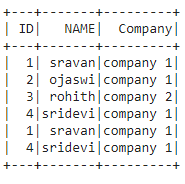Method 1: Using where() function

This function is used to check the condition and give the results

Syntax: dataframe.where(condition)

We are going to filter the rows by using column values through the condition, where the condition is the dataframe condition

Example 1: filter rows in dataframe where ID =1

## Python3

 `# get the data where ID=1 ` `dataframe.where(dataframe.``ID``=``=``'1'``).show() `

Output: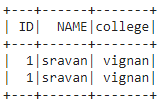Example 2:

## Python3

 `# get the data where name not 'sravan' ` `dataframe.where(dataframe.NAME !``=` `'sravan'``).show() `

Output: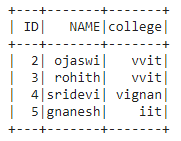Example 3: Where clause multiple column values filtering.

Python program to filter rows where ID greater than 2 and college is vvit

## Python3

 `# filter rows where ID greater than 2 ` `# and college is vvit ` `dataframe.where((dataframe.``ID``>``'2'``) & (dataframe.college``=``=``'vvit'``)).show() `

Output: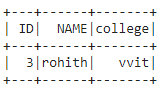Method 2: Using filter() function

This function is used to check the condition and give the results.

Syntax: dataframe.filter(condition)

Example 1: Python code to get column value = vvit college

## Python3

 `# get the data where college is  'vvit' ` `dataframe.``filter``(dataframe.college``=``=``'vvit'``).show() `

Output: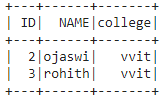Example 2: filter the data where id > 3.

## Python3

 `# get the data where id > 3 ` `dataframe.``filter``(dataframe.``ID``>``'3'``).show() `

Output: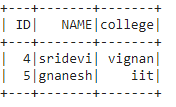Example 3: Multiple column value filtering.

Python program to filter rows where ID greater than 2 and college is vignan

## Python3

 `# filter rows where ID greater ` `# than 2 and college is vignan ` `dataframe.``filter``((dataframe.``ID``>``'2'``) & ` `                 ``(dataframe.college``=``=``'vignan'``)).show() `

Output: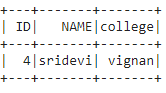Whether you're preparing for your first job interview or aiming to upskill in this ever-evolving tech landscape, GeeksforGeeks Courses are your key to success. We provide top-quality content at affordable prices, all geared towards accelerating your growth in a time-bound manner. Join the millions we've already empowered, and we're here to do the same for you. Don't miss out - check it out now!

Previous
Next